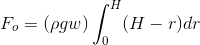# Wave paddle application Integration problem

## Homework Statement

This is an engineering design application, but It contains integration. Sorry if I didnt post it in the right folder.

I think a)'s answer should be (pgw)^2*h^2
h=r
To be honest no Idea what is going on..

Thanks

## Homework Statement

This is an engineering design application, but It contains integration. Sorry if I didnt post it in the right folder.

I think a)'s answer should be (pgw)^2*h^2
h=r
To be honest no Idea what is going on..

Thanks

What do the values 'p', 'g' and 'w' represent?

If they are just constants, then remove them from the integrand.
Eg.So, I think I've figured out 1a) ,b) ,c)
1a)
(pgwH^2)/2
1b)
Fo=-(pgwH^3)/3
1c)
θ=βsin(ωt)
dθ/dt=ωβcos(ωt)
d^2θ/dt=ω^2βsin(ωt)

Q2 gets something like:
T=-(pgwH^3)/3+Bωβcos(-tan^(-1)(Aω/B))-Aω^2βsin(tan^(-1)(Aω/B)
which seems a bit complicated to be integrated, because we have to find first its derivetive..
Thanks

haruspex
Homework Helper
Gold Member
2020 Award
1b)
Fo=-(pgwH^3)/3